# RD Sharma Solutions for Class 9 Maths Chapter 15 Area of Parallelograms and Triangles

## RD Sharma Solutions Class 9 Maths Chapter 15 – Free PDF Download

RD Sharma Solutions for Class 9 Maths Chapter 15 Areas Of Parallelograms And Triangles is a set of answers to all the exercise questions. In this chapter, students will study about Areas of Parallelograms and Triangles. Parallelogram, in Geometry, is a non-self-intersecting quadrilateral with two pairs of parallel sides. A trapezoid is a quadrilateral with only one pair of parallel sides. In a parallelogram, the diagonals bisect each other. Whereas, a triangle is a polygon with three vertices and three edges. A triangle can be classified into the isosceles triangle, equilateral triangle, and scalene triangle. The diagonal of a parallelogram are of equal lengths and each diagonal of a parallelogram separates it into two congruent triangles.

In this chapter, all the questions on triangles and parallelograms are formulated by expert teachers as per the CBSE 2021-22 syllabus. Students are advised to download the RD Sharma Class 9 Solutions PDF and start practicing to score good marks in the examinations. The RD Sharma Solutions for exercise-wise problems are prepared after vast research is conducted on each topic. The clear format of solutions helps students to solve problems with competence.

## Download PDF of RD Sharma Solutions for Class 9 Maths Chapter 15 Area of Parallelograms and Triangles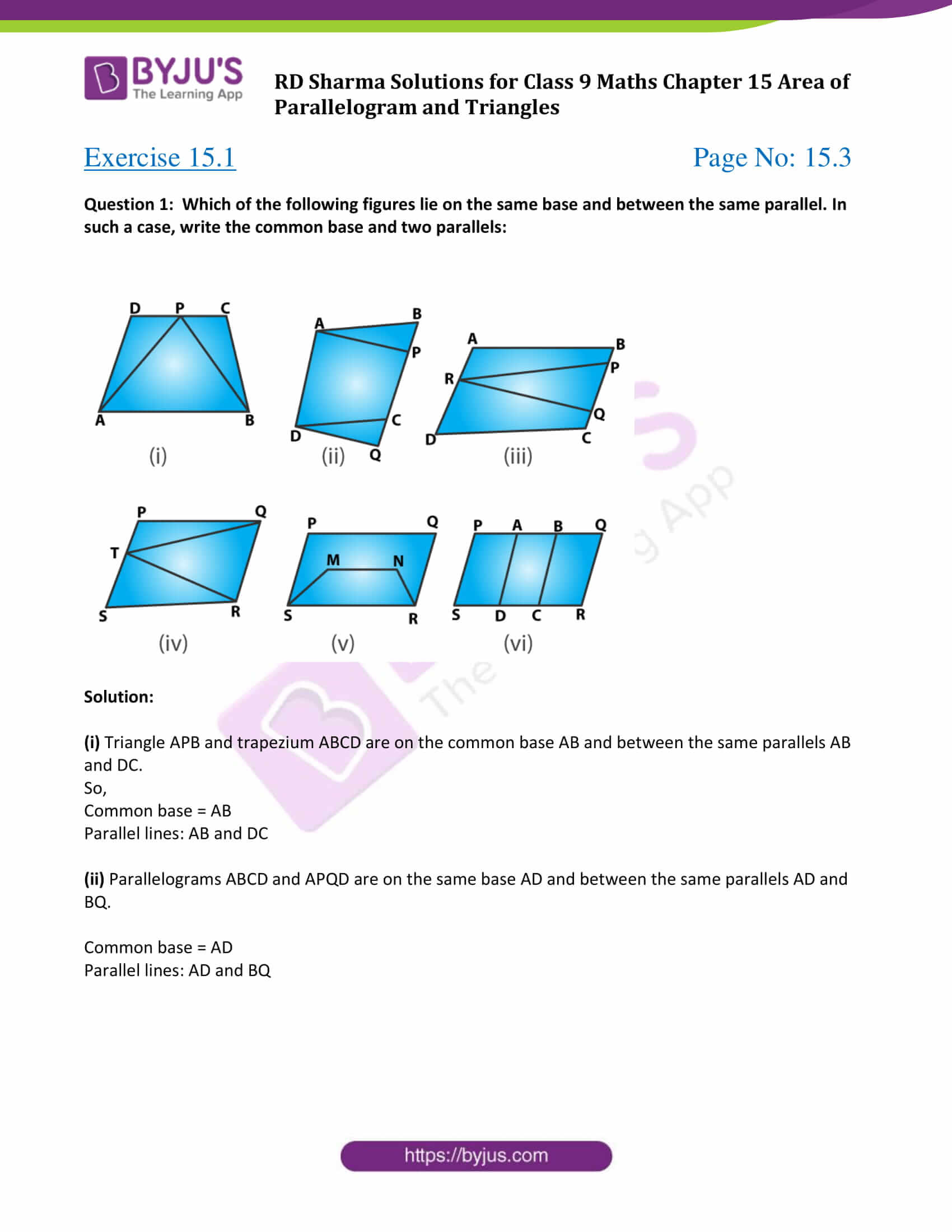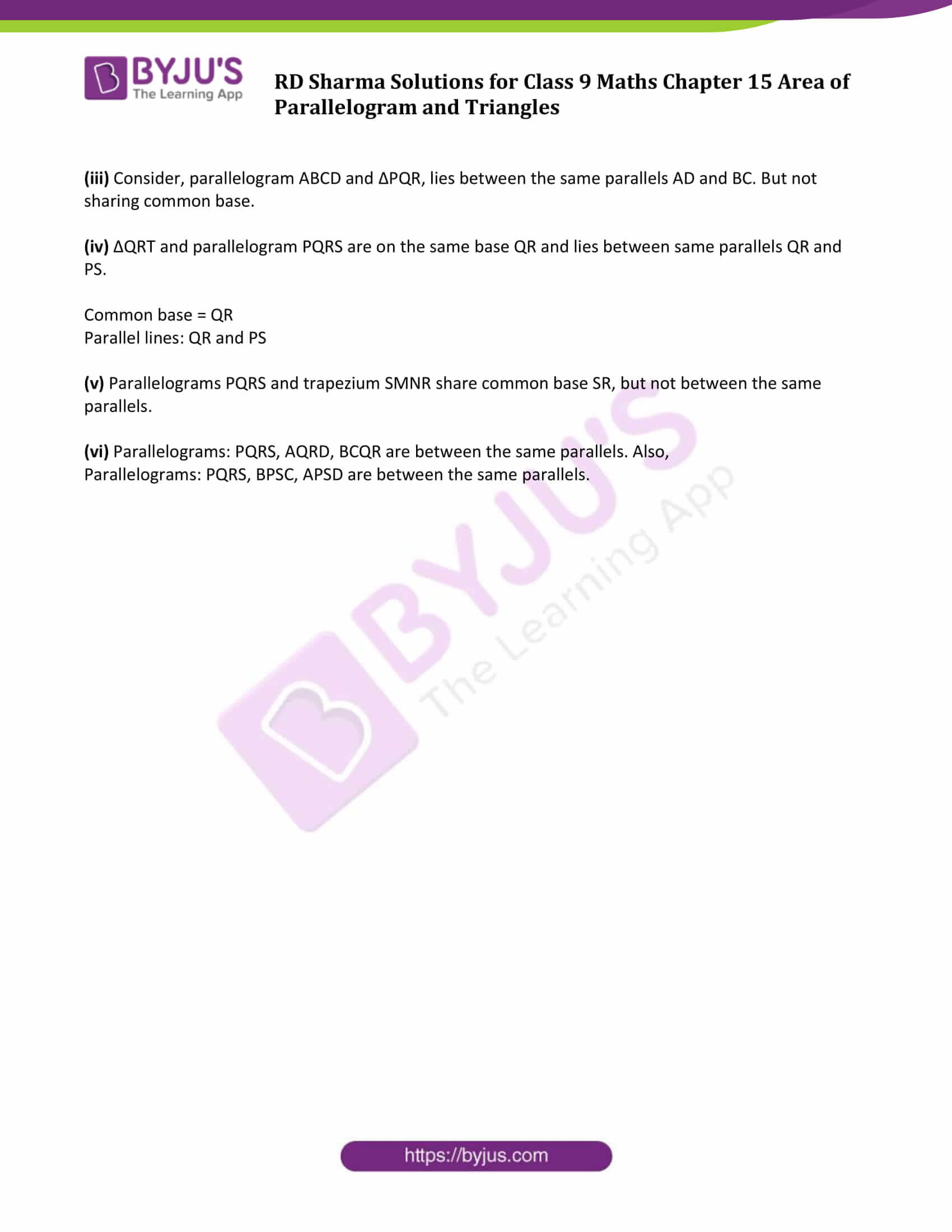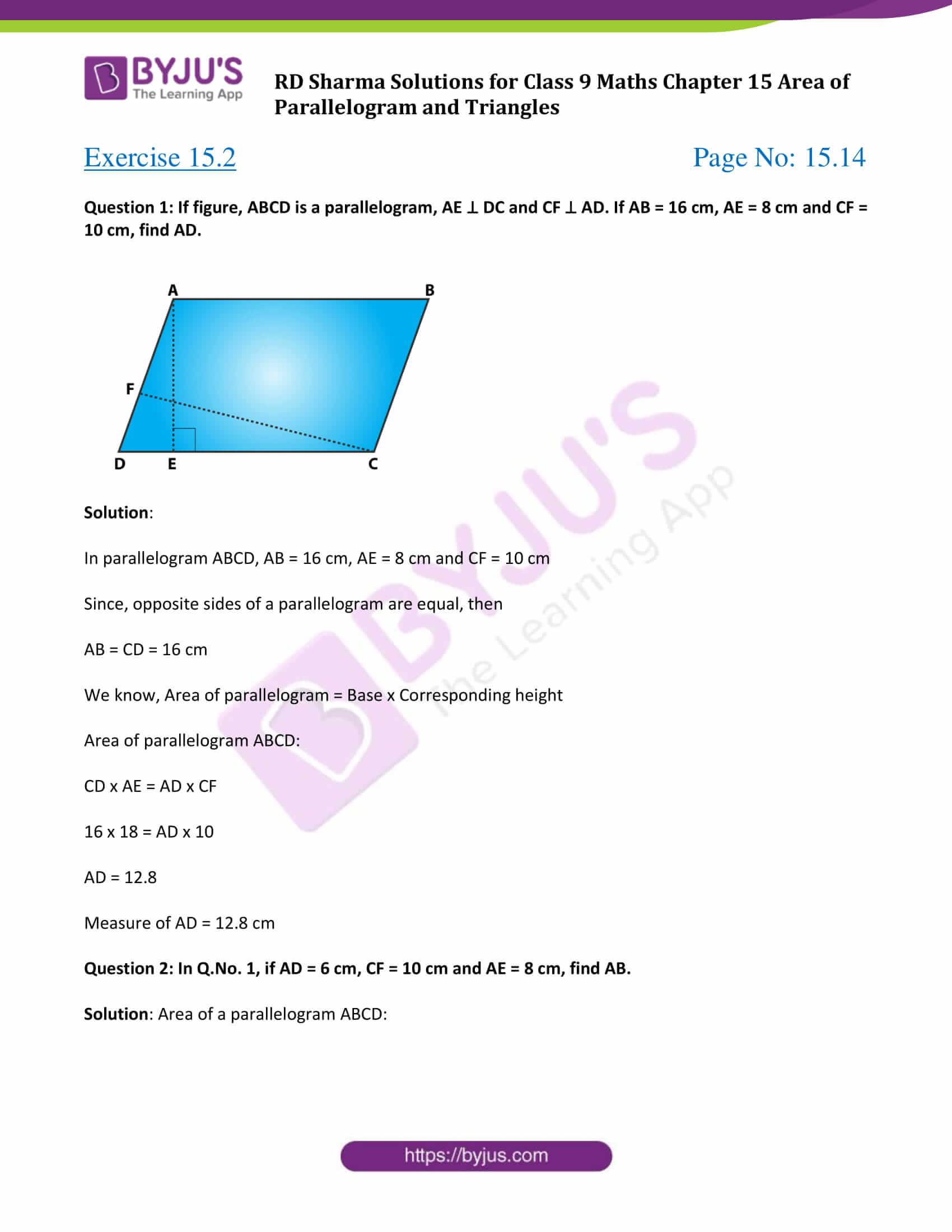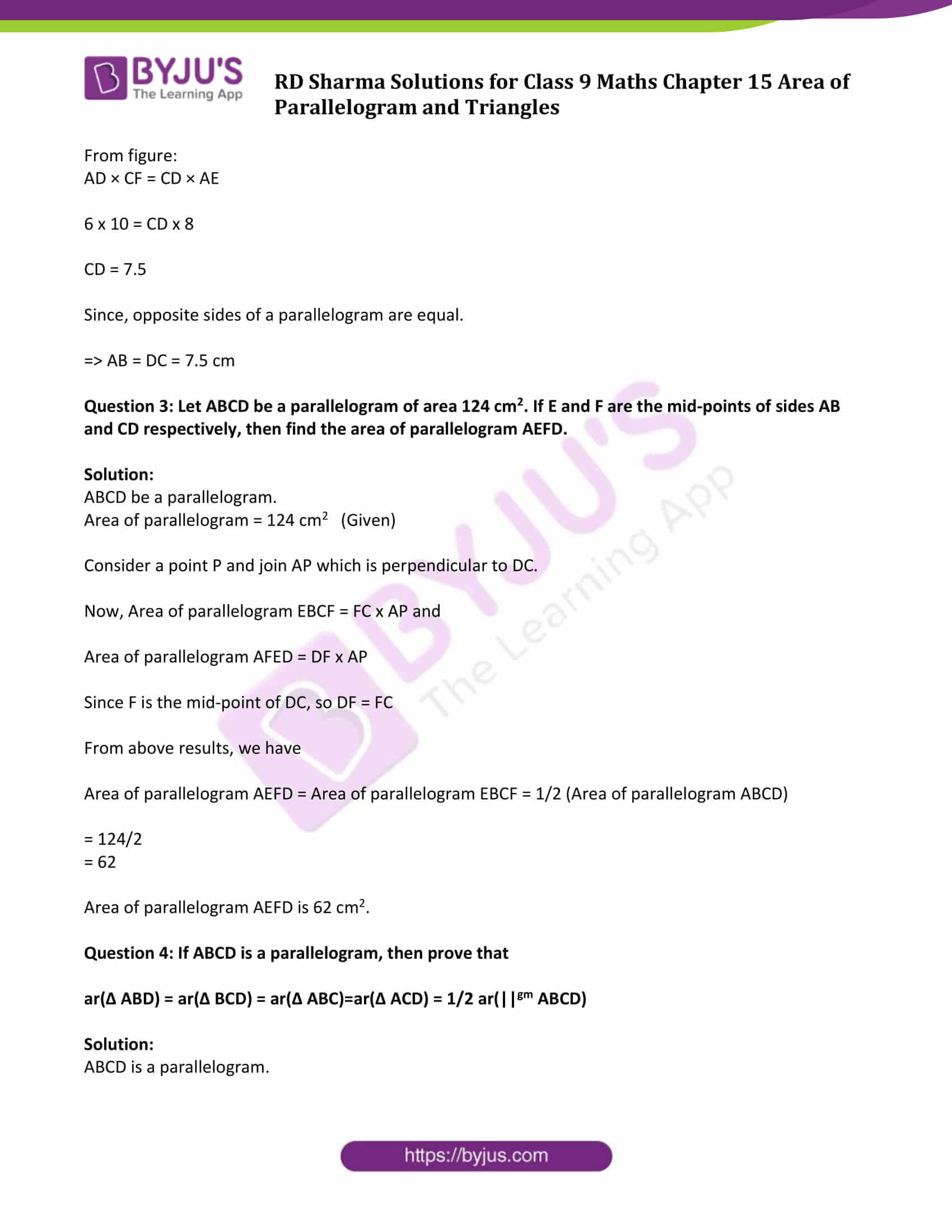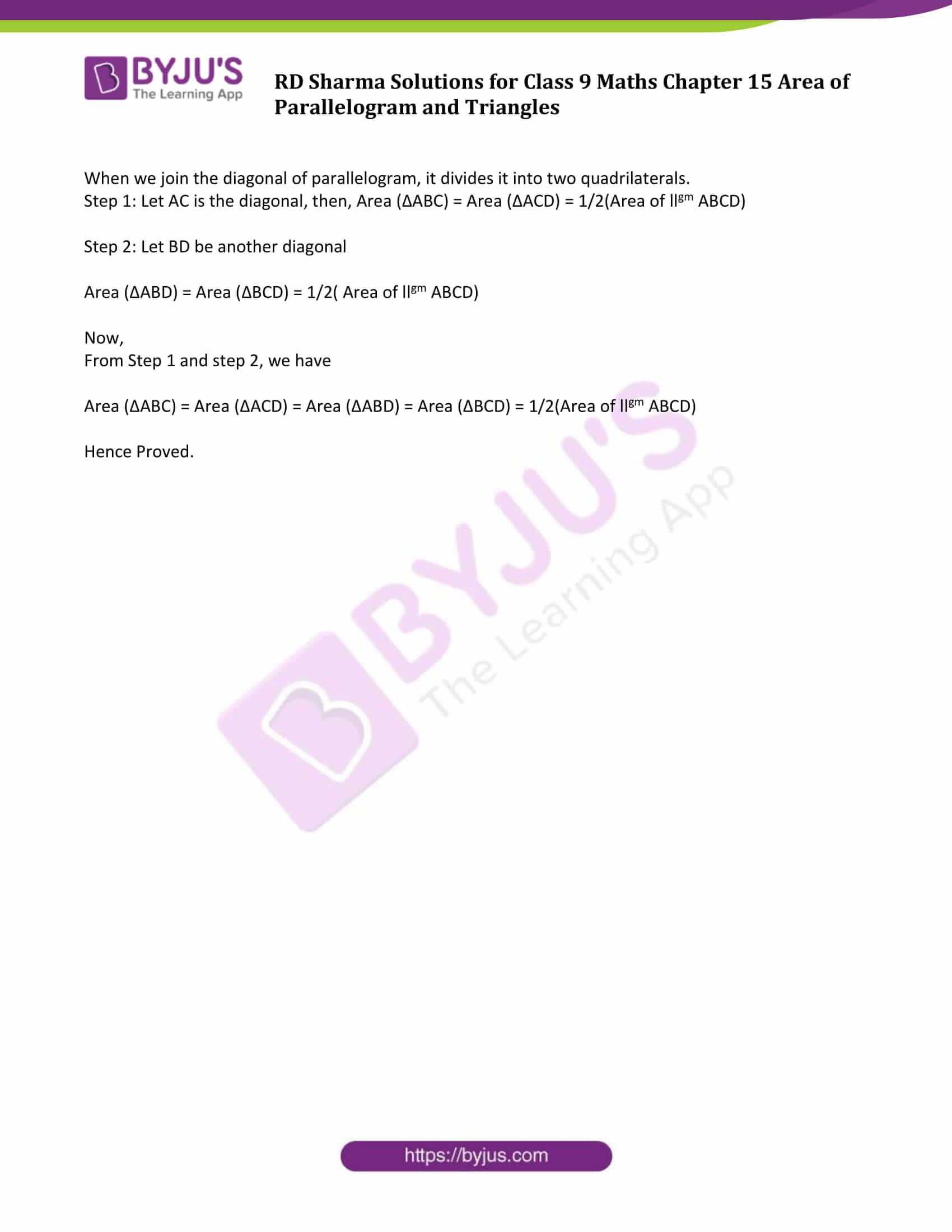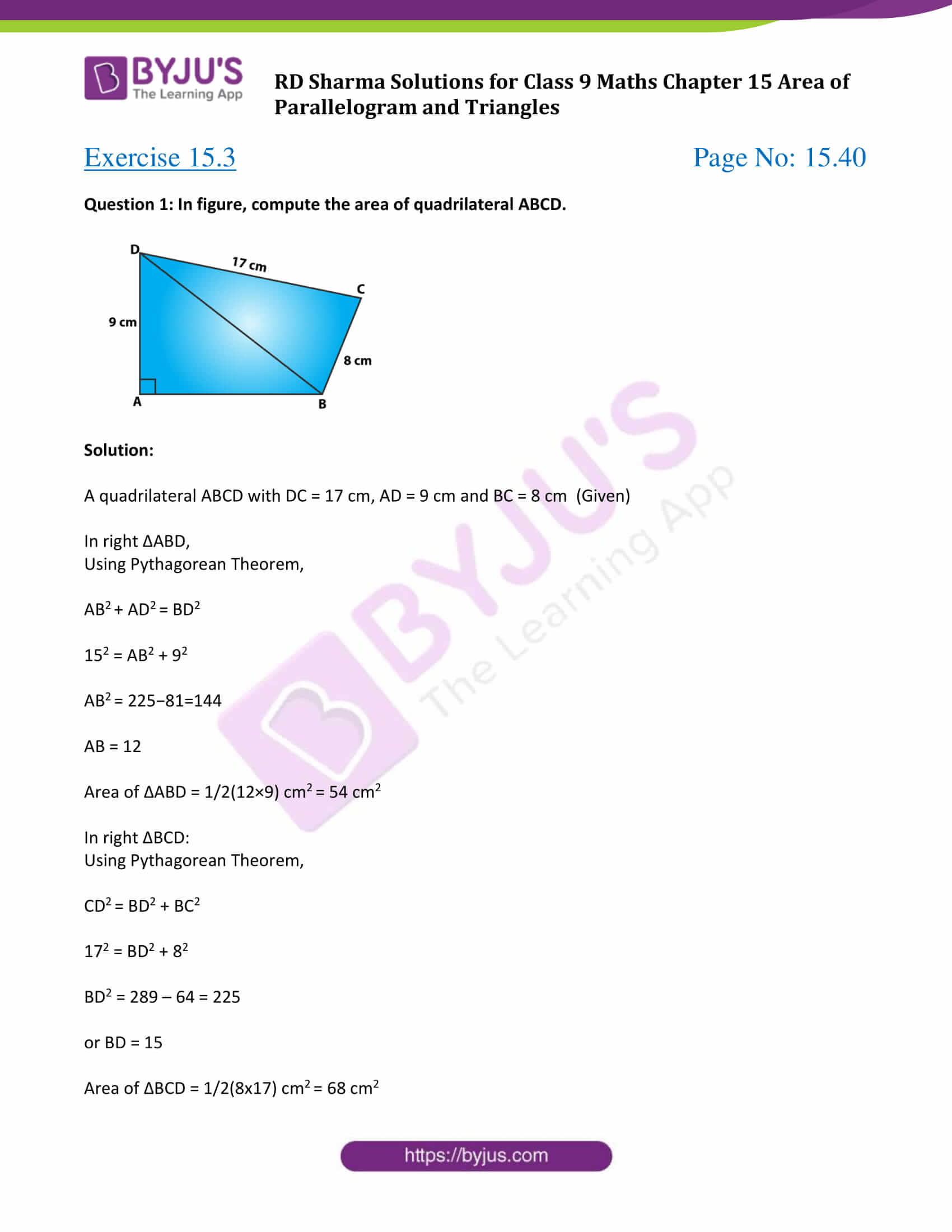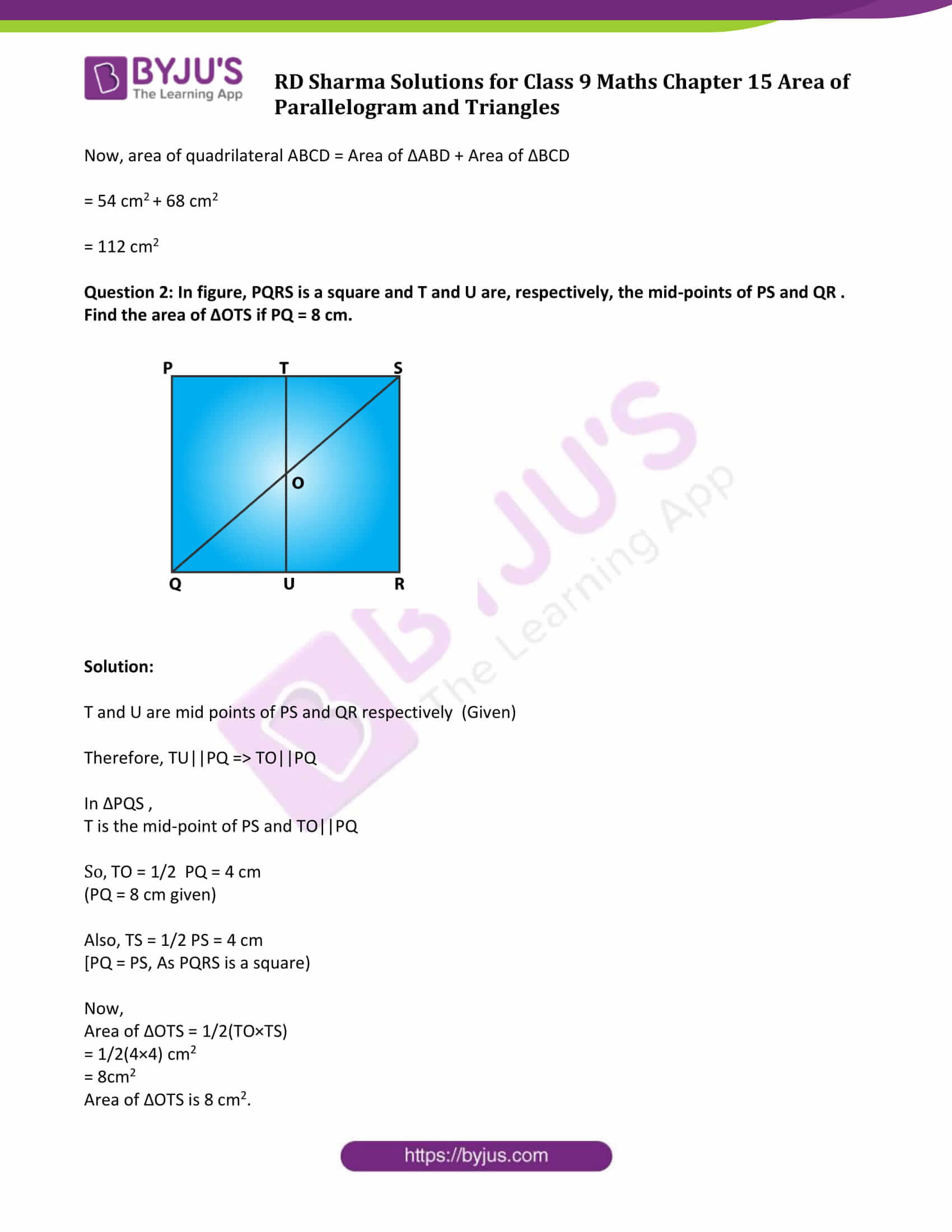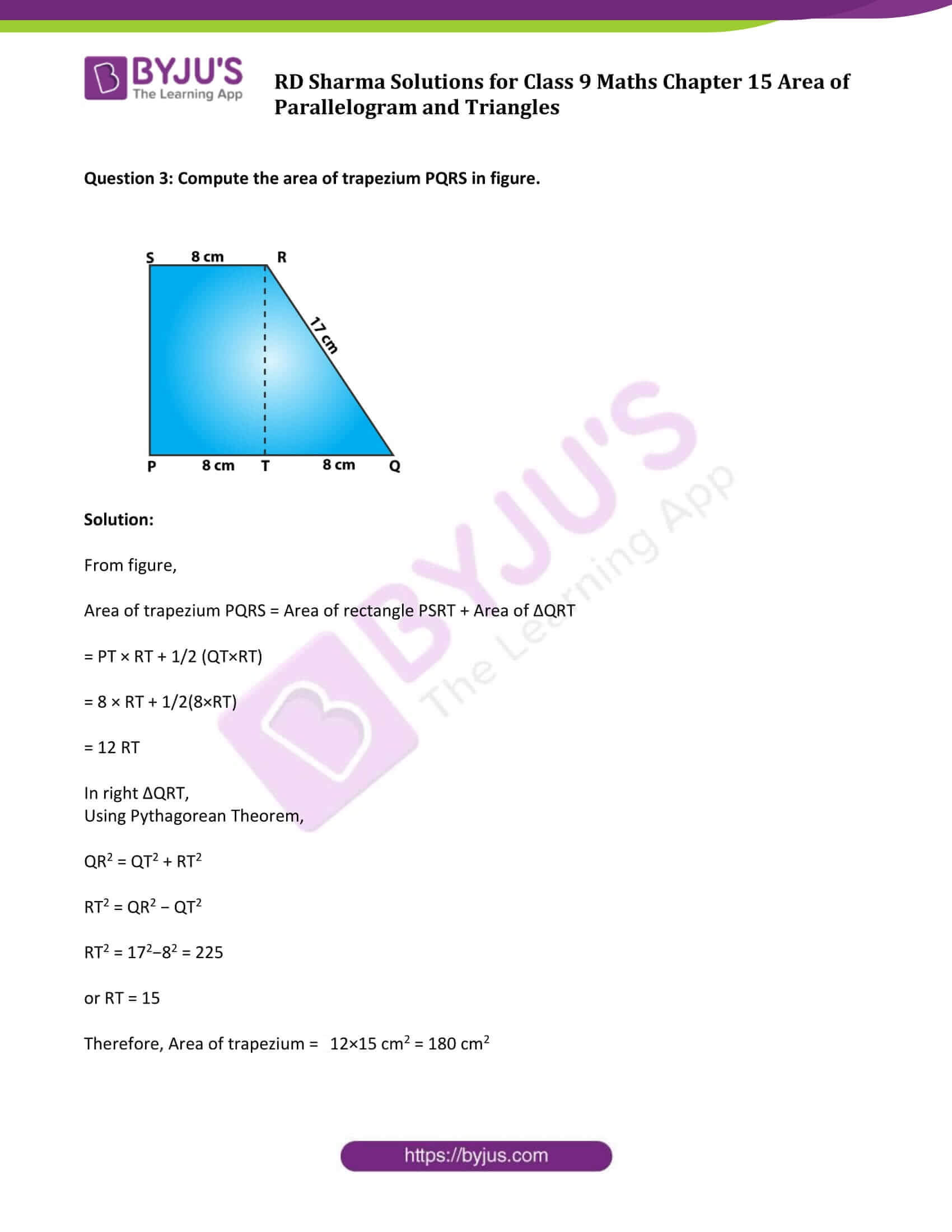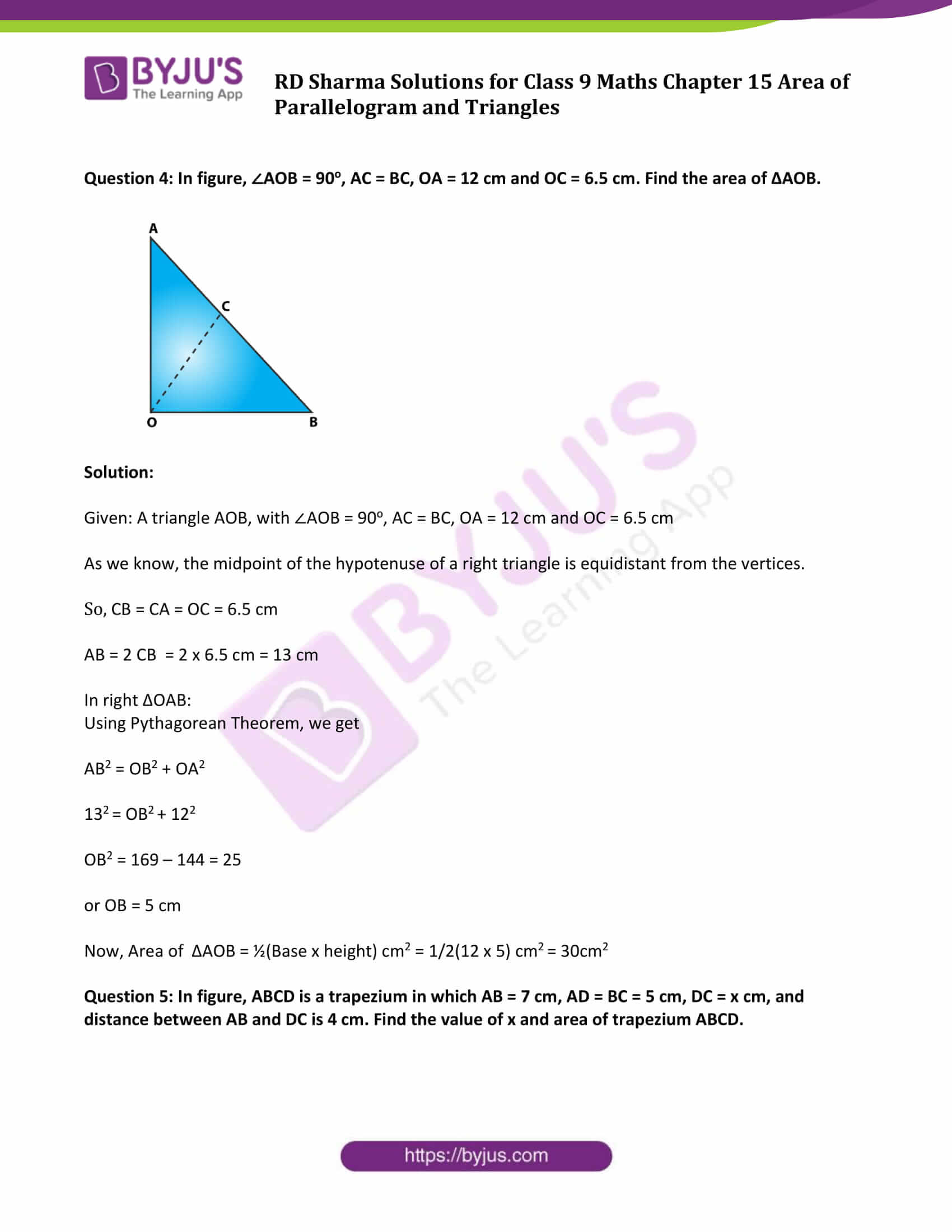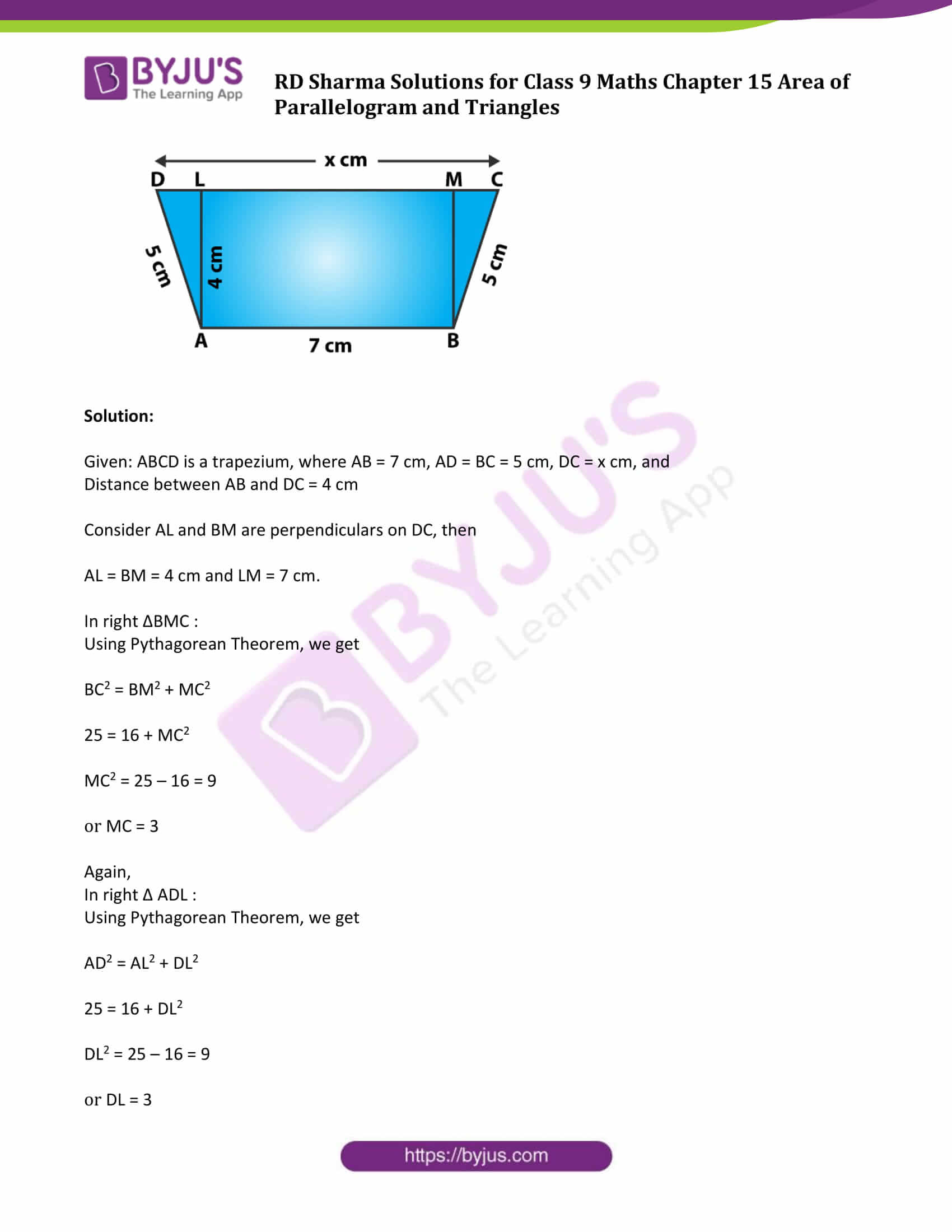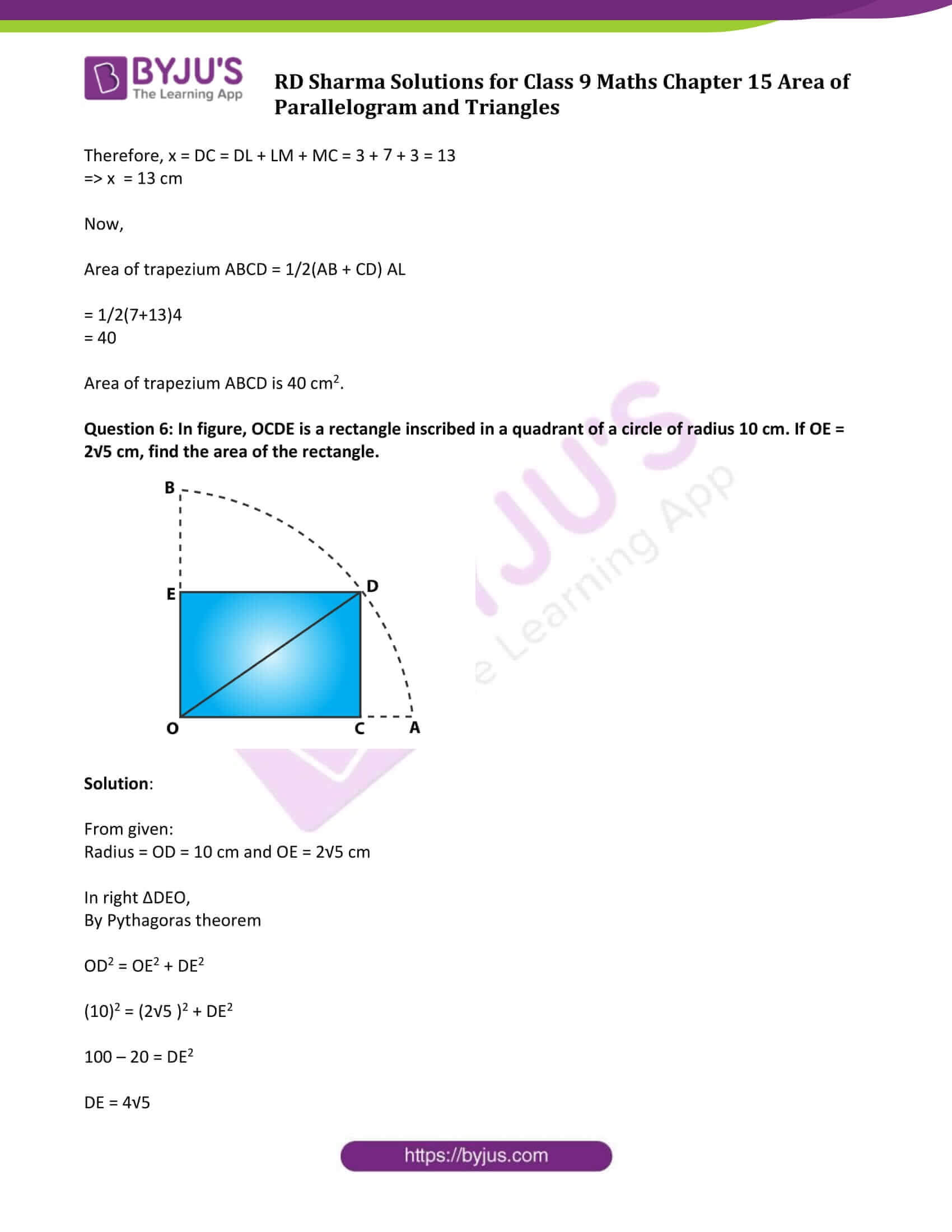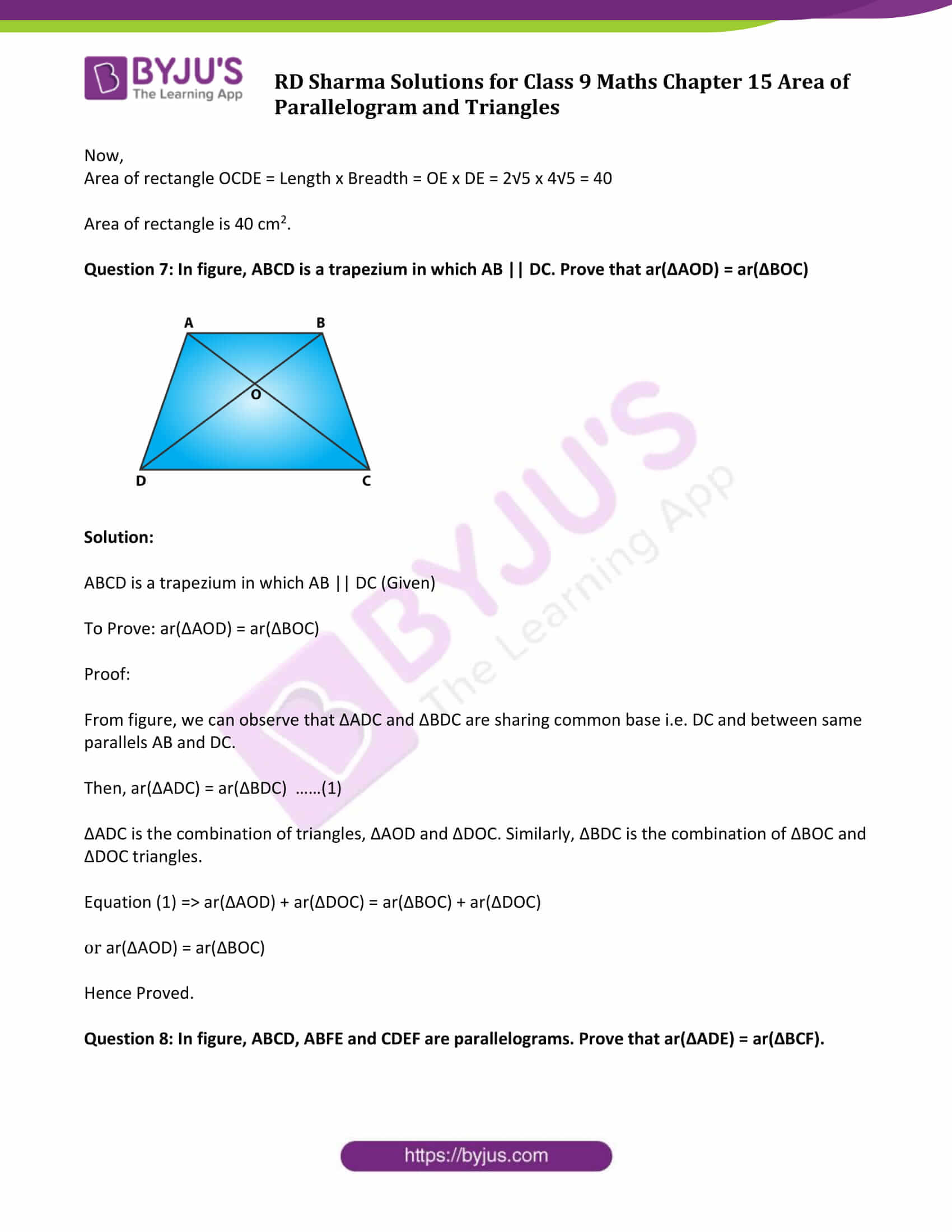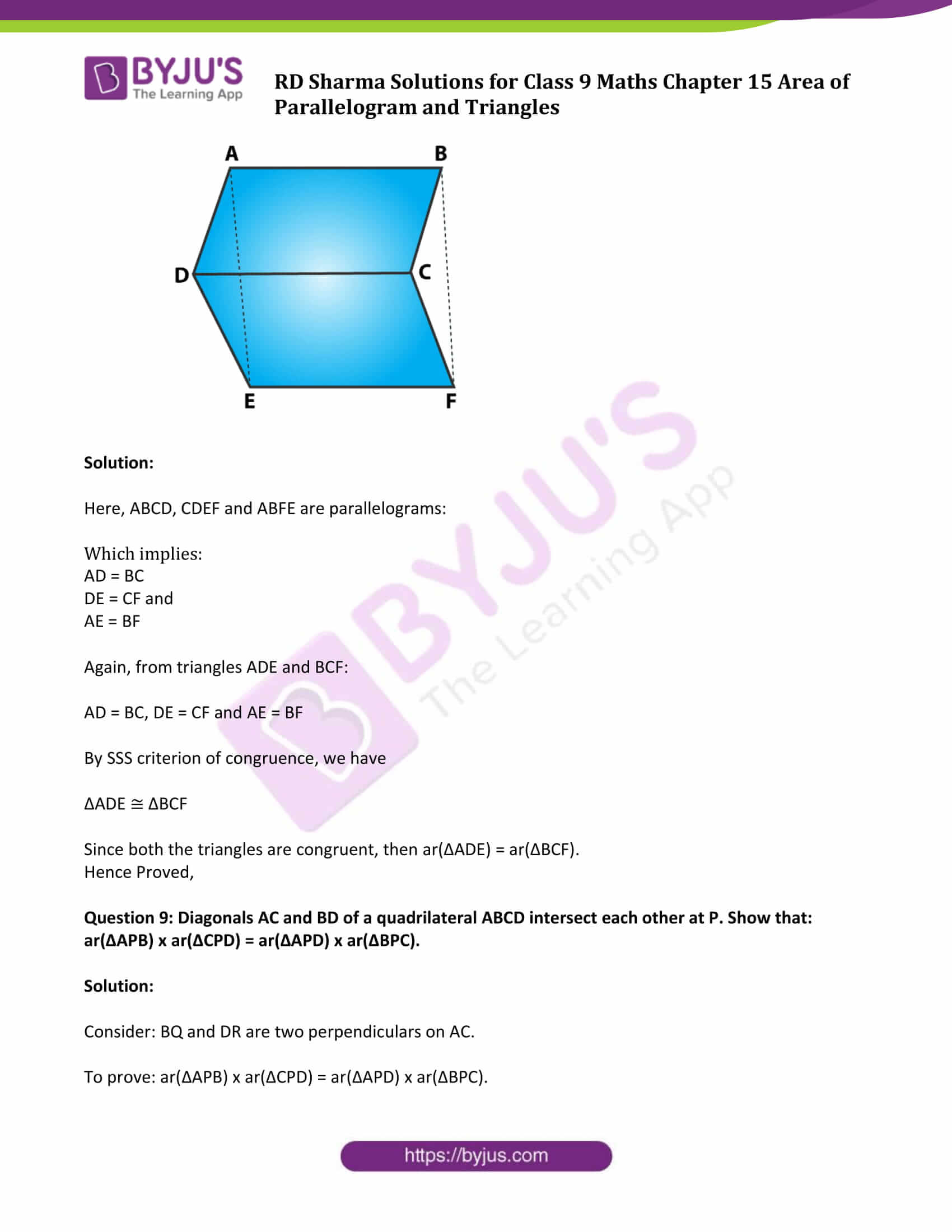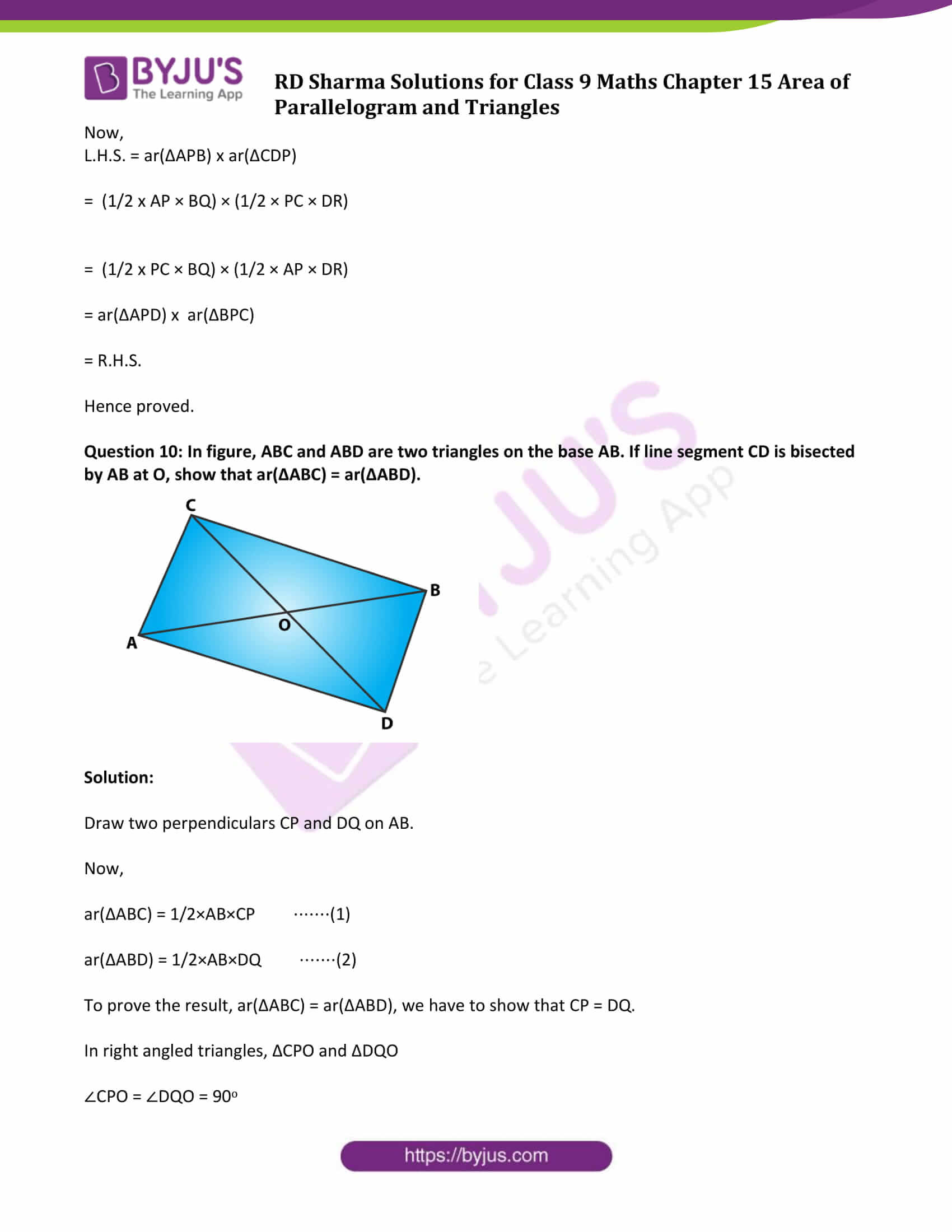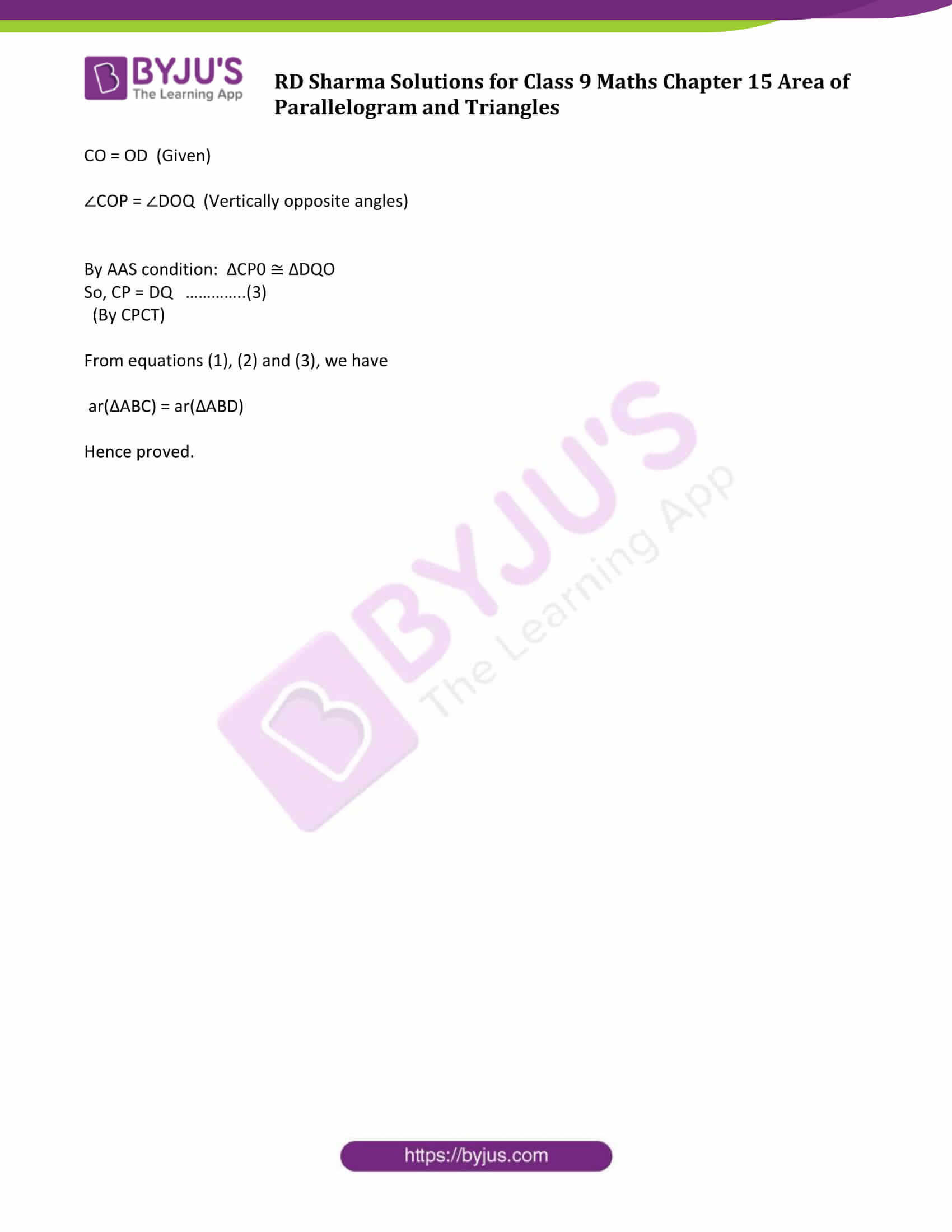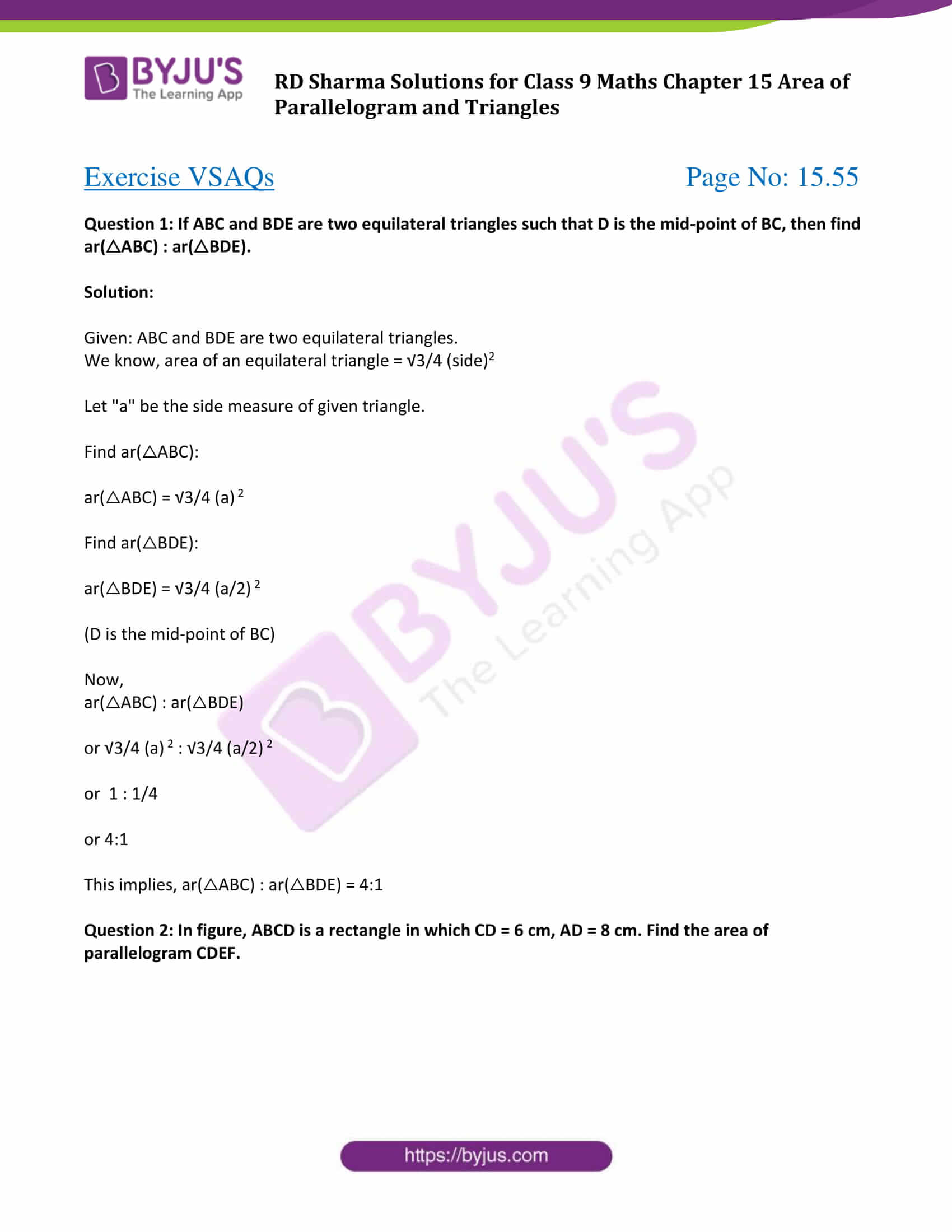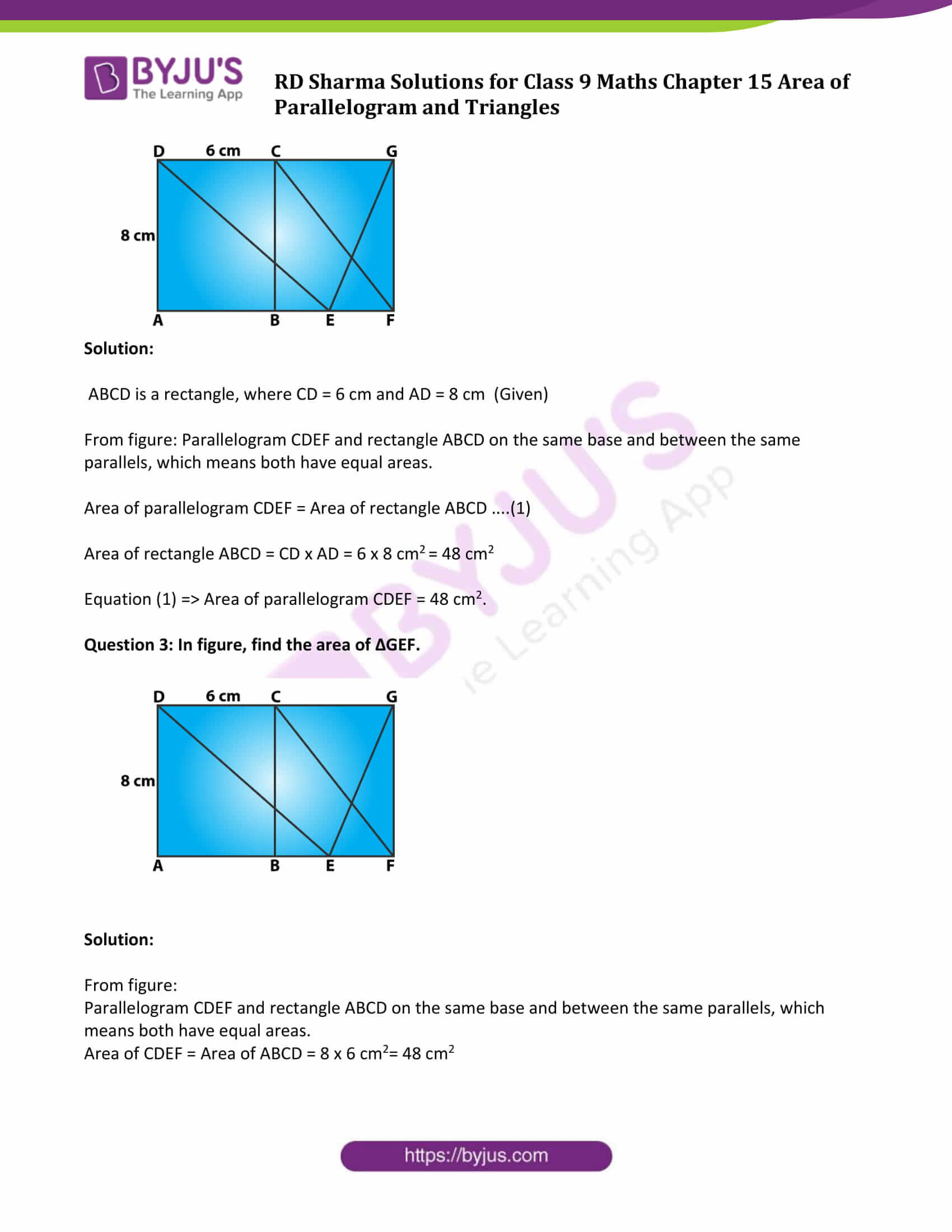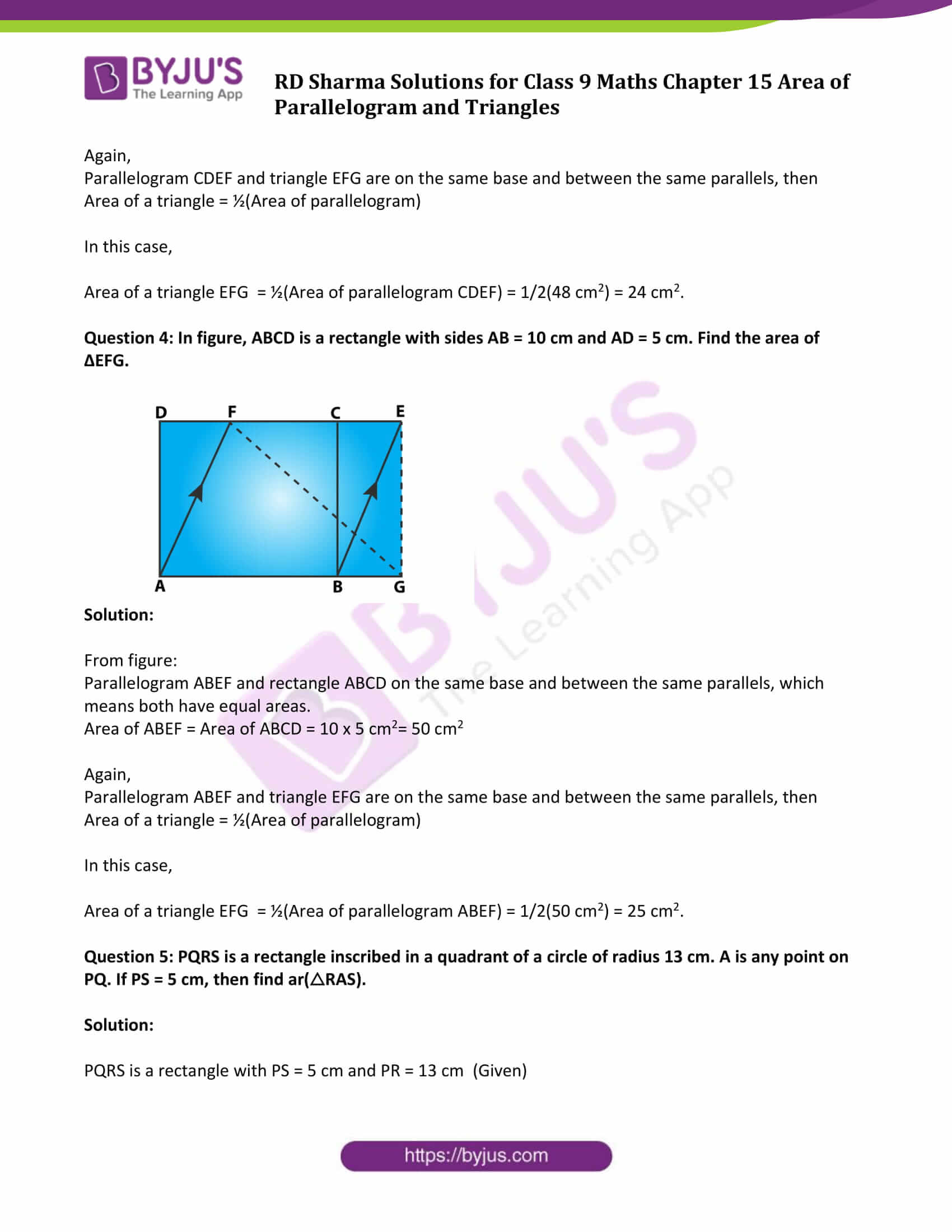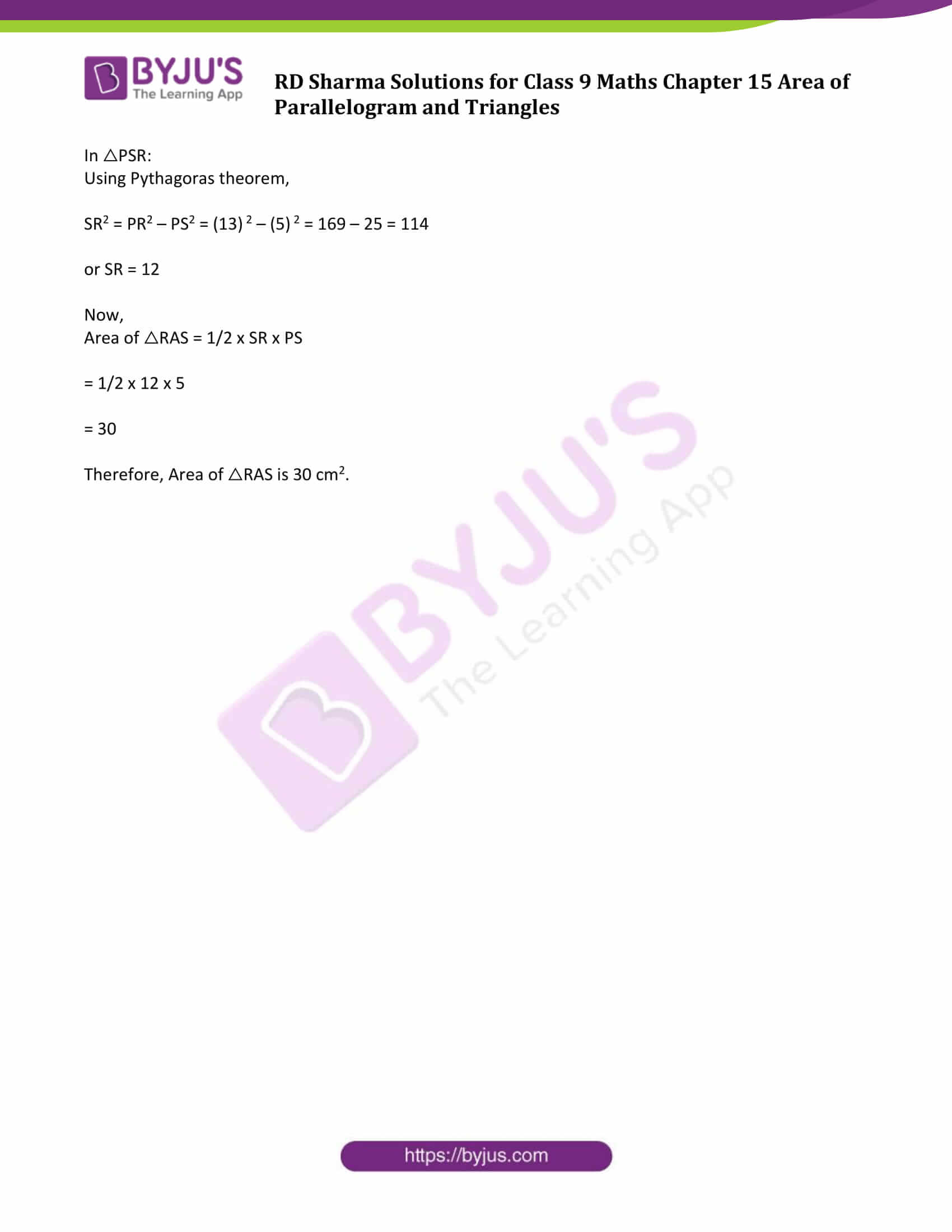### Exercise 15.1 Page No: 15.3

Question 1: Which of the following figures lie on the same base and between the same parallel. In such a case, write the common base and two parallels: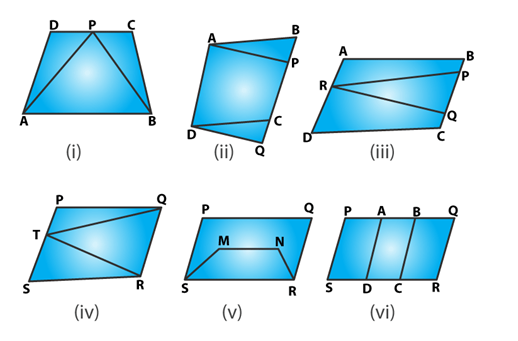Solution:

(i) Triangle APB and trapezium ABCD are on the common base AB and between the same parallels AB and DC.

So,

Common base = AB

Parallel lines: AB and DC

(ii) Parallelograms ABCD and APQD are on the same base AD and between the same parallels AD and BQ.

(iii) Consider, parallelogram ABCD and ΔPQR, lies between the same parallels AD and BC. But not sharing common base.

(iv) ΔQRT and parallelogram PQRS are on the same base QR and lies between same parallels QR and PS.

Common base = QR

Parallel lines: QR and PS

(v) Parallelograms PQRS and trapezium SMNR share common base SR, but not between the same parallels.

(vi) Parallelograms: PQRS, AQRD, BCQR are between the same parallels. Also,

Parallelograms: PQRS, BPSC, APSD are between the same parallels.

### Exercise 15.2 Page No: 15.14

Question 1: If figure, ABCD is a parallelogram, AE ⊥ DC and CF ⊥ AD. If AB = 16 cm, AE = 8 cm and CF = 10 cm, find AD.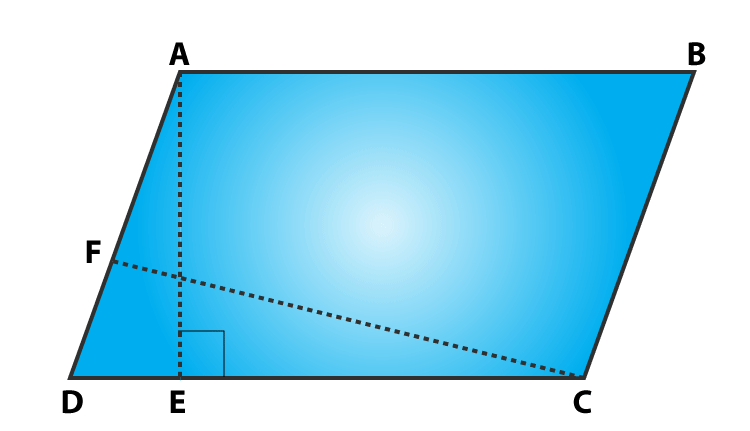Solution:

In parallelogram ABCD, AB = 16 cm, AE = 8 cm and CF = 10 cm

Since, opposite sides of a parallelogram are equal, then

AB = CD = 16 cm

We know, Area of parallelogram = Base x Corresponding height

Area of parallelogram ABCD:

CD x AE = AD x CF

16 x 18 = AD x 10

Measure of AD = 12.8 cm

Question 2: In Q.No. 1, if AD = 6 cm, CF = 10 cm and AE = 8 cm, find AB.

Solution: Area of a parallelogram ABCD:

From figure:

AD × CF = CD × AE

6 x 10 = CD x 8

CD = 7.5

Since, opposite sides of a parallelogram are equal.

=> AB = DC = 7.5 cm

Question 3: Let ABCD be a parallelogram of area 124 cm2. If E and F are the mid-points of sides AB and CD respectively, then find the area of parallelogram AEFD.

Solution:

ABCD be a parallelogram.

Area of parallelogram = 124 cm2 (Given)

Consider a point P and join AP which is perpendicular to DC.

Now, Area of parallelogram EBCF = FC x AP and

Area of parallelogram AFED = DF x AP

Since F is the mid-point of DC, so DF = FC

From above results, we have

Area of parallelogram AEFD = Area of parallelogram EBCF = 1/2 (Area of parallelogram ABCD)

= 124/2

= 62

Area of parallelogram AEFD is 62 cm2.

Question 4: If ABCD is a parallelogram, then prove that

ar(Δ ABD) = ar(Δ BCD) = ar(Δ ABC)=ar(Δ ACD) = 1/2 ar(||gm ABCD)

Solution:

ABCD is a parallelogram.

When we join the diagonal of parallelogram, it divides it into two quadrilaterals.

Step 1: Let AC is the diagonal, then, Area (ΔABC) = Area (ΔACD) = 1/2(Area of llgm ABCD)

Step 2: Let BD be another diagonal

Area (ΔABD) = Area (ΔBCD) = 1/2( Area of llgm ABCD)

Now,

From Step 1 and step 2, we have

Area (ΔABC) = Area (ΔACD) = Area (ΔABD) = Area (ΔBCD) = 1/2(Area of llgm ABCD)

Hence Proved.

### Exercise 15.3 Page No: 15.40

Question 1: In figure, compute the area of quadrilateral ABCD.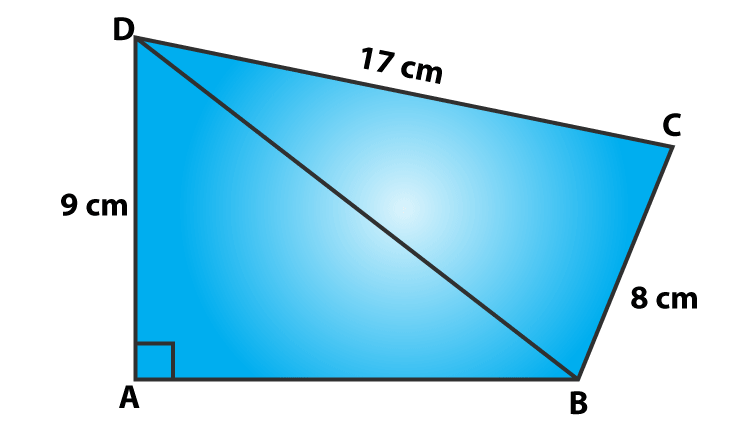Solution:

A quadrilateral ABCD with DC = 17 cm, AD = 9 cm and BC = 8 cm (Given)

In right ΔABD,

Using Pythagorean Theorem,

152 = AB2 + 92

AB2 = 225−81=144

AB = 12

Area of ΔABD = 1/2(12×9) cm2 = 54 cm2

In right ΔBCD:

Using Pythagorean Theorem,

CD2 = BD2 + BC2

172 = BD2 + 82

BD2 = 289 – 64 = 225

or BD = 15

Area of ΔBCD = 1/2(8×17) cm2 = 68 cm2

Now, area of quadrilateral ABCD = Area of ΔABD + Area of ΔBCD

= 54 cm2 + 68 cm2

= 112 cm2

Question 2: In figure, PQRS is a square and T and U are, respectively, the mid-points of PS and QR . Find the area of ΔOTS if PQ = 8 cm.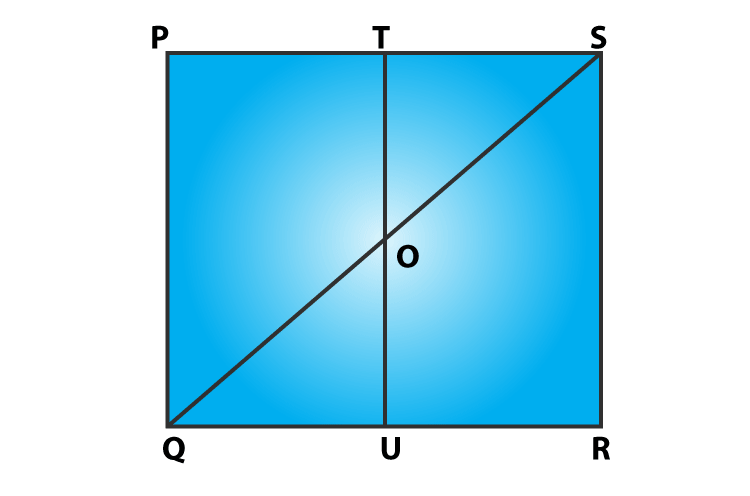Solution:

T and U are mid points of PS and QR respectively (Given)

Therefore, TU||PQ => TO||PQ

In ΔPQS ,

T is the mid-point of PS and TO||PQ

So, TO = 1/2 PQ = 4 cm

(PQ = 8 cm given)

Also, TS = 1/2 PS = 4 cm

[PQ = PS, As PQRS is a square)

Now,

Area of ΔOTS = 1/2(TO×TS)

= 1/2(4×4) cm2

= 8cm2

Area of ΔOTS is 8 cm2.

Question 3: Compute the area of trapezium PQRS in figure.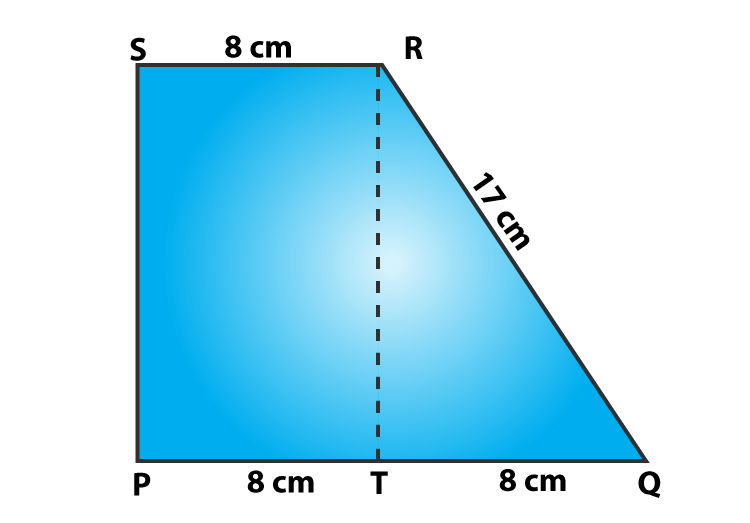Solution:

From figure,

Area of trapezium PQRS = Area of rectangle PSRT + Area of ΔQRT

= PT × RT + 1/2 (QT×RT)

= 8 × RT + 1/2(8×RT)

= 12 RT

In right ΔQRT,

Using Pythagorean Theorem,

QR2 = QT2 + RT2

RT2 = QR2 − QT2

RT2 = 172−82 = 225

or RT = 15

Therefore, Area of trapezium = 12×15 cm2 = 180 cm2

Question 4: In figure, ∠AOB = 90o, AC = BC, OA = 12 cm and OC = 6.5 cm. Find the area of ΔAOB.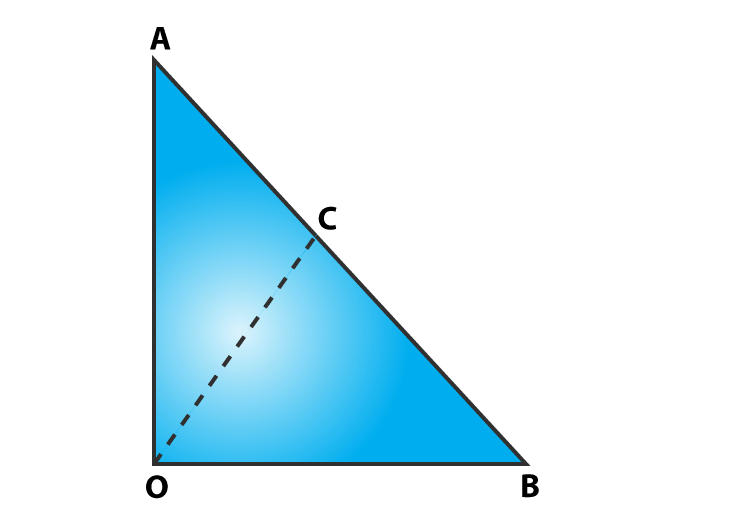Solution:

Given: A triangle AOB, with ∠AOB = 90o, AC = BC, OA = 12 cm and OC = 6.5 cm

As we know, the midpoint of the hypotenuse of a right triangle is equidistant from the vertices.

So, CB = CA = OC = 6.5 cm

AB = 2 CB = 2 x 6.5 cm = 13 cm

In right ΔOAB:

Using Pythagorean Theorem, we get

AB2 = OB2 + OA2

132 = OB2 + 122

OB2 = 169 – 144 = 25

or OB = 5 cm

Now, Area of ΔAOB = ½(Base x height) cm2 = 1/2(12 x 5) cm2 = 30cm2

Question 5: In figure, ABCD is a trapezium in which AB = 7 cm, AD = BC = 5 cm, DC = x cm, and distance between AB and DC is 4 cm. Find the value of x and area of trapezium ABCD.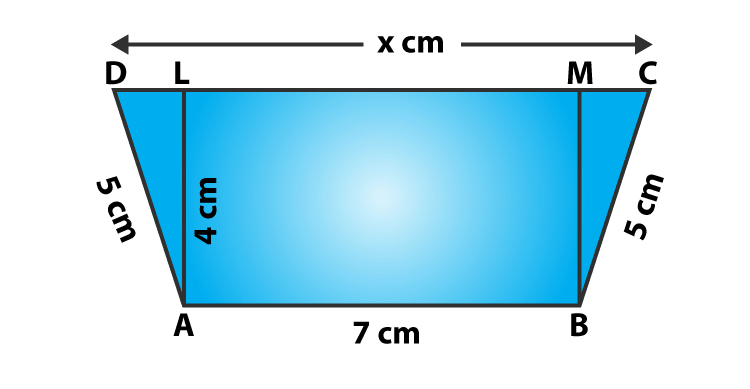Solution:

Given: ABCD is a trapezium, where AB = 7 cm, AD = BC = 5 cm, DC = x cm, and

Distance between AB and DC = 4 cm

Consider AL and BM are perpendiculars on DC, then

AL = BM = 4 cm and LM = 7 cm.

In right ΔBMC :

Using Pythagorean Theorem, we get

BC2 = BM2 + MC2

25 = 16 + MC2

MC2 = 25 – 16 = 9

or MC = 3

Again,

Using Pythagorean Theorem, we get

25 = 16 + DL2

DL2 = 25 – 16 = 9

or DL = 3

Therefore, x = DC = DL + LM + MC = 3 + 7 + 3 = 13

=> x = 13 cm

Now,

Area of trapezium ABCD = 1/2(AB + CD) AL

= 1/2(7+13)4

= 40

Area of trapezium ABCD is 40 cm2.

Question 6: In figure, OCDE is a rectangle inscribed in a quadrant of a circle of radius 10 cm. If OE = 2√5 cm, find the area of the rectangle.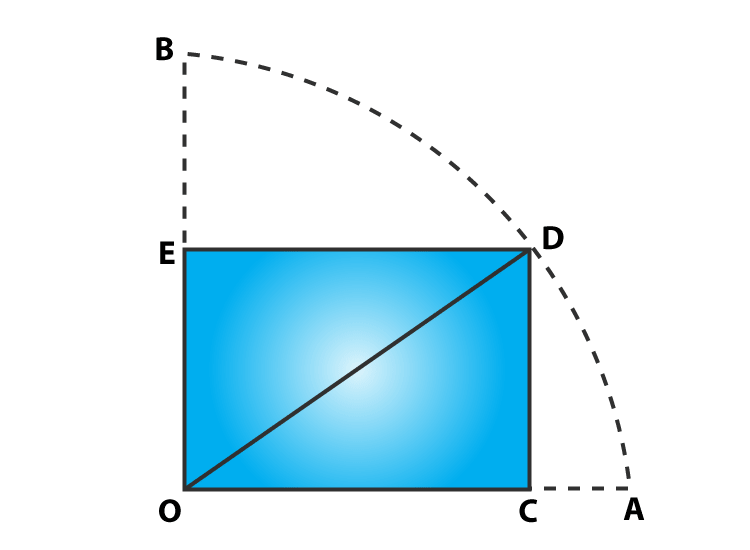Solution:

From given:

Radius = OD = 10 cm and OE = 2√5 cm

In right ΔDEO,

By Pythagoras theorem

OD2 = OE2 + DE2

(10)2 = (2√5 )2 + DE2

100 – 20 = DE2

DE = 4√5

Now,

Area of rectangle OCDE = Length x Breadth = OE x DE = 2√5 x 4√5 = 40

Area of rectangle is 40 cm2.

Question 7: In figure, ABCD is a trapezium in which AB || DC. Prove that ar(ΔAOD) = ar(ΔBOC)Solution:

ABCD is a trapezium in which AB || DC (Given)

To Prove: ar(ΔAOD) = ar(ΔBOC)

Proof:

From figure, we can observe that ΔADC and ΔBDC are sharing common base i.e. DC and between same parallels AB and DC.

ΔADC is the combination of triangles, ΔAOD and ΔDOC. Similarly, ΔBDC is the combination of ΔBOC and ΔDOC triangles.

Equation (1) => ar(ΔAOD) + ar(ΔDOC) = ar(ΔBOC) + ar(ΔDOC)

or ar(ΔAOD) = ar(ΔBOC)

Hence Proved.

Question 8: In figure, ABCD, ABFE and CDEF are parallelograms. Prove that ar(ΔADE) = ar(ΔBCF).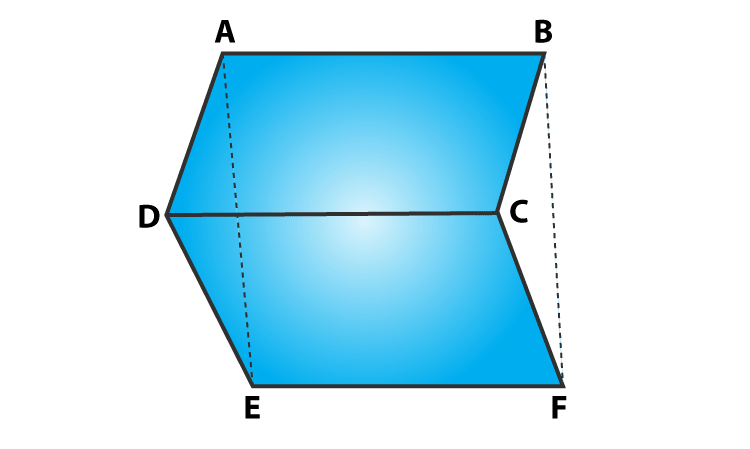Solution:

Here, ABCD, CDEF and ABFE are parallelograms:

Which implies:

DE = CF and

AE = BF

Again, from triangles ADE and BCF:

AD = BC, DE = CF and AE = BF

By SSS criterion of congruence, we have

Since both the triangles are congruent, then ar(ΔADE) = ar(ΔBCF).

Hence Proved,

Question 9: Diagonals AC and BD of a quadrilateral ABCD intersect each other at P. Show that: ar(ΔAPB) x ar(ΔCPD) = ar(ΔAPD) x ar(ΔBPC).

Solution:

Consider: BQ and DR are two perpendiculars on AC.

To prove: ar(ΔAPB) x ar(ΔCPD) = ar(ΔAPD) x ar(ΔBPC).

Now,

L.H.S. = ar(ΔAPB) x ar(ΔCDP)

= (1/2 x AP × BQ) × (1/2 × PC × DR)

= (1/2 x PC × BQ) × (1/2 × AP × DR)

= ar(ΔAPD) x ar(ΔBPC)

= R.H.S.

Hence proved.

Question 10: In figure, ABC and ABD are two triangles on the base AB. If line segment CD is bisected by AB at O, show that ar(ΔABC) = ar(ΔABD).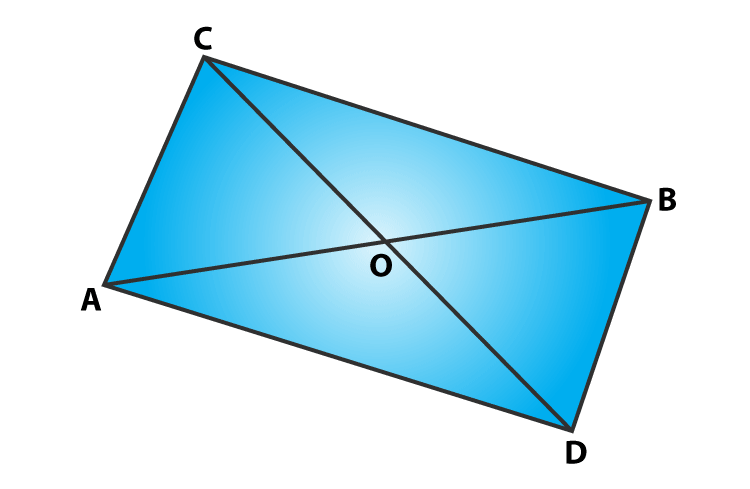Solution:

Draw two perpendiculars CP and DQ on AB.

Now,

ar(ΔABC) = 1/2×AB×CP ⋅⋅⋅⋅⋅⋅⋅(1)

ar(ΔABD) = 1/2×AB×DQ ⋅⋅⋅⋅⋅⋅⋅(2)

To prove the result, ar(ΔABC) = ar(ΔABD), we have to show that CP = DQ.

In right angled triangles, ΔCPO and ΔDQO

∠CPO = ∠DQO = 90o

CO = OD (Given)

∠COP = ∠DOQ (Vertically opposite angles)

By AAS condition: ΔCP0 ≅ ΔDQO

So, CP = DQ …………..(3)

(By CPCT)

From equations (1), (2) and (3), we have

ar(ΔABC) = ar(ΔABD)

Hence proved.

### Exercise VSAQs Page No: 15.55

Question 1: If ABC and BDE are two equilateral triangles such that D is the mid-point of BC, then find ar(△ABC) : ar(△BDE).

Solution:

Given: ABC and BDE are two equilateral triangles.

We know, area of an equilateral triangle = √3/4 (side)2

Let “a” be the side measure of given triangle.

Find ar(△ABC):

ar(△ABC) = √3/4 (a) 2

Find ar(△BDE):

ar(△BDE) = √3/4 (a/2) 2

(D is the mid-point of BC)

Now,

ar(△ABC) : ar(△BDE)

or √3/4 (a) 2 : √3/4 (a/2) 2

or 1 : 1/4

or 4:1

This implies, ar(△ABC) : ar(△BDE) = 4:1

Question 2: In figure, ABCD is a rectangle in which CD = 6 cm, AD = 8 cm. Find the area of parallelogram CDEF.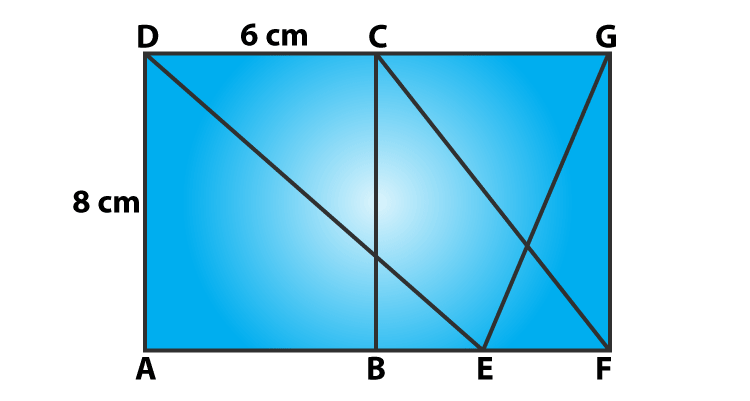Solution:

ABCD is a rectangle, where CD = 6 cm and AD = 8 cm (Given)

From figure: Parallelogram CDEF and rectangle ABCD on the same base and between the same parallels, which means both have equal areas.

Area of parallelogram CDEF = Area of rectangle ABCD ….(1)

Area of rectangle ABCD = CD x AD = 6 x 8 cm2 = 48 cm2

Equation (1) => Area of parallelogram CDEF = 48 cm2.

Question 3: In figure, find the area of ΔGEF.Solution:

From figure:

Parallelogram CDEF and rectangle ABCD on the same base and between the same parallels, which means both have equal areas.

Area of CDEF = Area of ABCD = 8 x 6 cm2= 48 cm2

Again,

Parallelogram CDEF and triangle EFG are on the same base and between the same parallels, then

Area of a triangle = ½(Area of parallelogram)

In this case,

Area of a triangle EFG = ½(Area of parallelogram CDEF) = 1/2(48 cm2) = 24 cm2.

Question 4: In figure, ABCD is a rectangle with sides AB = 10 cm and AD = 5 cm. Find the area of ΔEFG.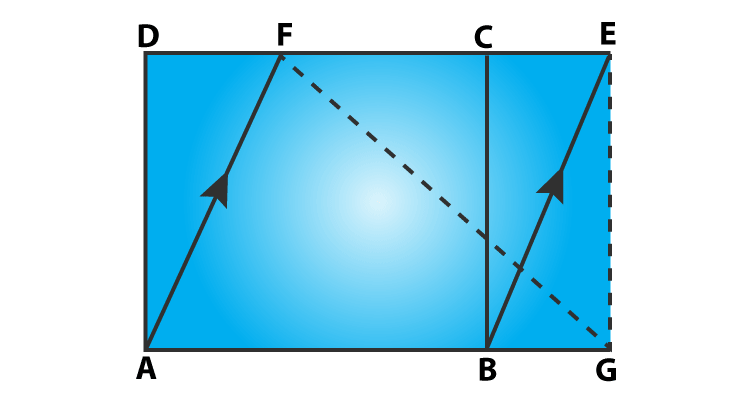Solution:

From figure:

Parallelogram ABEF and rectangle ABCD on the same base and between the same parallels, which means both have equal areas.

Area of ABEF = Area of ABCD = 10 x 5 cm2= 50 cm2

Again,

Parallelogram ABEF and triangle EFG are on the same base and between the same parallels, then

Area of a triangle = ½(Area of parallelogram)

In this case,

Area of a triangle EFG = ½(Area of parallelogram ABEF) = 1/2(50 cm2) = 25 cm2.

Question 5: PQRS is a rectangle inscribed in a quadrant of a circle of radius 13 cm. A is any point on PQ. If PS = 5 cm, then find ar(△RAS).

Solution:

PQRS is a rectangle with PS = 5 cm and PR = 13 cm (Given)

In △PSR:

Using Pythagoras theorem,

SR2 = PR2 – PS2 = (13) 2 – (5) 2 = 169 – 25 = 114

or SR = 12

Now,

Area of △RAS = 1/2 x SR x PS

= 1/2 x 12 x 5

= 30

Therefore, Area of △RAS is 30 cm2.

## RD Sharma Solutions for Class 9 Maths Chapter 15 Area of Parallelograms and Triangles

In the 15th Chapter of Class 9,  RD Sharma Solutions students will study important concepts. Some are listed below:

• Area of Parallelograms and Triangles Introduction.
• Figures on the same base and between the same parallels
• Geometric figures Regions
• Area Axioms
• Parallelogram on the same base and between the same parallels

### Key benefits of studying RD Sharma books

• RD Sharma books provide several questions to obtain confidence in solving complex problems with ease. This develops critical thinking and strengthens the mental ability of the students.
• These solutions facilitate the students to have a good knowledge of basic as well as advanced mathematical concepts. It also helps students in retaining and quickly retrieving the concepts.
• The illustrations provided in RD Sharma books are offered in a step by step approach for a comfortable and better understanding of concepts. The accurate answers also boost to grasp the methods of solving the problems effortlessly.
• These solutions are the best resource materials for students who aspire to gain proficiency in the subject and score good marks in academics.

## Frequently Asked Questions on RD Sharma Solutions for Class 9 Maths Chapter 15

### Why RD Sharma Solutions for Class 9 Maths Chapter 15 is important from an exam point of view?

RD Sharma Solutions for Class 9 Maths Chapter 15 Areas of Parallelogram and Triangles consist of important questions which help to secure high marks. The concepts present in this chapter are essential not only for board exams but also for other competitive exams as well. Subject matter experts explained the concepts in a simple and easy manner so that students understand them more effectively.

### Is it necessary to practice all the questions provided in RD Sharma Solutions for Class 9 Maths Chapter 15?

Yes, practising all the questions provided in RD Sharma Solutions for Class 9 Maths Chapter 15 boosts skills required to secure more marks in exams. These solutions are provided in simple language so that students solve the problems without any difficulty and grasp the concepts in-depth. Subject experts designed the solutions in a descriptive manner as per the current CBSE board.

### How does RD Sharma Solutions for Class 9 Maths Chapter 15 Areas of Parallelogram and Triangles boost exam preparation among students?

RD Sharma Solutions for Class 9 Maths Chapter 15 are solved in a detailed manner with illustrations to help students in effective exam preparation. The solutions prepared by experts clear the doubts immediately and boost conceptual knowledge among students. By practising these solutions on a regular basis, students not only grasp the concepts thoroughly but also procure more marks in board exams.